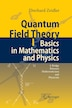# Quantum Field Theory I: Basics In Mathematics And Physics: A Bridge Between Mathematicians And Physicists

## byEberhard Zeidler

### Paperback | August 23, 2016\$220.95
Earn 1,105 plum® points
Prices and offers may vary in store
Quantity:

In stock online

Ships free on orders over \$35

Not available in stores

1 Daß ich erkenne, was die Welt im Innersten zusammenh¨ alt. Faust Concepts without intuition are empty, intuition without concepts is blind. Immanuel Kant (1724-1804) The greatest mathematicians like Archimedes, Newton, and Gauss have always been able to combine theory and applications into one. Felix Klein (1849-1925) The present comprehensive introduction to the mathematical and physical aspects of quantum ?eld theory consists of the following six volumes: Volume I: Basics in Mathematics and Physics Volume II: Quantum Electrodynamics Volume III: Gauge Theory Volume IV: Quantum Mathematics Volume V: The Physics of the Standard Model Volume VI: Quantum Gravity and String Theory. Since ancient times, both physicists and mathematicians have tried to und- stand the forces acting in nature. Nowadays we know that there exist four fundamental forces in nature: . Newton's gravitational force, . Maxwell's electromagnetic force, . the strong force between elementary particles, and . the weak force between elementary particles (e.g., the force responsible for the radioactive decay of atoms). In the 20th century, physicists established two basic models, namely, . the Standard Model in cosmology based on Einstein's theory of general relativity, and . the Standard Model in elementary particle physics based on gauge theory. 1 So that I may perceive whatever holds the world together in its inmost folds.
Preface.- Part I. Introduction.- Prologue.- 1. Historical Introduction.- 2. Phenomenology of the Standard Model for Elementary Particles.- 3. The Challenge of Different Scales in Nature.- Part II. Basic Techniques in Mathematics.- 4. Analyticity.- 5. A Glance at Topology.- 6. Many-Particle Systems in Mathematics and Physics.- 7. Rigoro...